While defining the cross product in geometric terms, then it is possible to gain understanding on properties of cross product. But, for computation it is necessary to get acquainted with formula which is not possible to understand through geometric definition. The calculation of cross product of two vectors is possible through this formula:

a x b = |a| |b| sin (θ) n

• |a| = magnitude of vector a
• |b| =magnitude of vector b
• n = unit vector
• Θ = angle between A and B

Therefore, the length says about the length of A times and the length of B times and finally the sine of angle between A and B. We finally multiply by vector n and ensure that the head is in right direction.

Properties of cross product:

1. The length of cross product of two vector is determined as”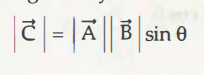1. Anti-commutativity: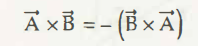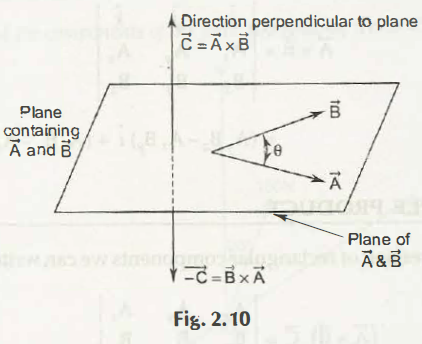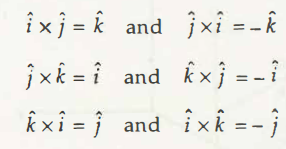1. Multiplication by scalars: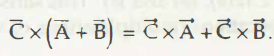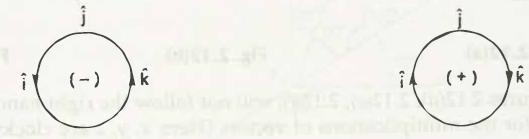1. Distributivity: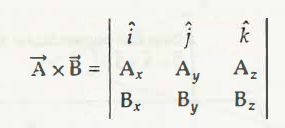1. The scalar product of vectors a, b and c

Links of Next Mechanical Engineering Topics:-### Customer Reviews

My Homework Help
Rated 5.0 out of 5 based on 510 customer reviews at